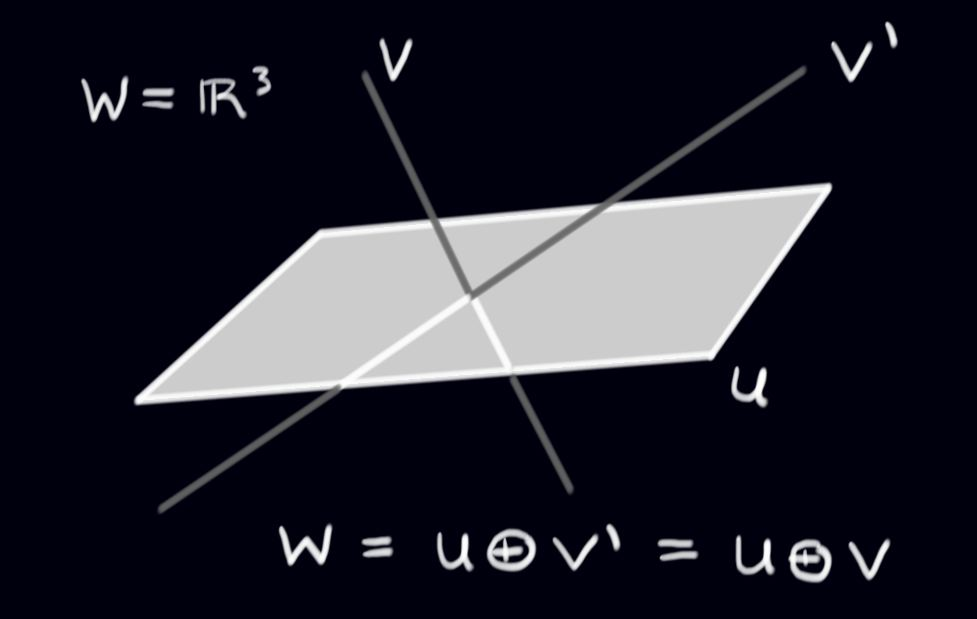$$\newcommand{\id}{\mathrm{id}}$$ $$\newcommand{\Span}{\mathrm{span}}$$ $$\newcommand{\kernel}{\mathrm{null}\,}$$ $$\newcommand{\range}{\mathrm{range}\,}$$ $$\newcommand{\RealPart}{\mathrm{Re}}$$ $$\newcommand{\ImaginaryPart}{\mathrm{Im}}$$ $$\newcommand{\Argument}{\mathrm{Arg}}$$ $$\newcommand{\norm}{\| #1 \|}$$ $$\newcommand{\inner}{\langle #1, #2 \rangle}$$ $$\newcommand{\Span}{\mathrm{span}}$$

# 14.6: Orthogonal Complements

$$\newcommand{\vecs}{\overset { \rightharpoonup} {\mathbf{#1}} }$$ $$\newcommand{\vecd}{\overset{-\!-\!\rightharpoonup}{\vphantom{a}\smash {#1}}}$$$$\newcommand{\id}{\mathrm{id}}$$ $$\newcommand{\Span}{\mathrm{span}}$$ $$\newcommand{\kernel}{\mathrm{null}\,}$$ $$\newcommand{\range}{\mathrm{range}\,}$$ $$\newcommand{\RealPart}{\mathrm{Re}}$$ $$\newcommand{\ImaginaryPart}{\mathrm{Im}}$$ $$\newcommand{\Argument}{\mathrm{Arg}}$$ $$\newcommand{\norm}{\| #1 \|}$$ $$\newcommand{\inner}{\langle #1, #2 \rangle}$$ $$\newcommand{\Span}{\mathrm{span}}$$ $$\newcommand{\id}{\mathrm{id}}$$ $$\newcommand{\Span}{\mathrm{span}}$$ $$\newcommand{\kernel}{\mathrm{null}\,}$$ $$\newcommand{\range}{\mathrm{range}\,}$$ $$\newcommand{\RealPart}{\mathrm{Re}}$$ $$\newcommand{\ImaginaryPart}{\mathrm{Im}}$$ $$\newcommand{\Argument}{\mathrm{Arg}}$$ $$\newcommand{\norm}{\| #1 \|}$$ $$\newcommand{\inner}{\langle #1, #2 \rangle}$$ $$\newcommand{\Span}{\mathrm{span}}$$

Let $$U$$ and $$V$$ be subspaces of a vector space $$W$$. In review exercise 6 you are asked to show that $$U\cap V$$ is a subspace of $$W$$, and that $$U\cup V$$ is not a subspace. However, $$span (U\cup V)$$ is certainly a subspace, since the span of $$\textit{any}$$ subset of a vector space is a subspace. Notice that all elements of $$span (U\cup V)$$ take the form $$u+v$$ with $$u\in U$$ and $$v\in V$$. We call the subspace $$U+V:=span (U\cup V) = \{u+v | u\in U, v\in V \}$$ the $$\textit{sum}$$ of $$U$$ and $$V$$. Here, we are not adding vectors, but vector spaces to produce a new vector space!

Definition: Direct sum

Given two subspaces $$U$$ and $$V$$ of a space $$W$$ such that $$U\cap V=\{0_{W}\}\, ,$$ the $$\textit{direct sum}$$ of $$U$$ and $$V$$ is defined as:

$U \oplus V = span (U\cup V)= \{u+v | u\in U, v\in V \}.$

Remark: When $$U\cap V= \{0_{W}\}$$, $$U+V=U\oplus V$$.

The direct sum has a very nice property:

Theorem

If $$w\in U\oplus V$$ then the expression $$w=u+v$$ is unique. That is, there is only one way to write $$w$$ as the sum of a vector in $$U$$ and a vector in $$V$$.

Proof

Suppose that $$u+v=u'+v'$$, with $$u,u'\in U$$, and $$v,v' \in V$$. Then we could express $$0=(u-u')+(v-v')$$. Then $$(u-u')=-(v-v')$$. Since $$U$$ and $$V$$ are subspaces, we have $$(u-u')\in U$$ and $$-(v-v')\in V$$. But since these elements are equal, we also have $$(u-u')\in V$$. Since $$U\cap V=\{0\}$$, then $$(u-u')=0$$. Similarly, $$(v-v')=0$$. Therefore $$u=u'$$ and $$v=v'$$, proving the theorem.

Given a subspace $$U$$ in $$W$$, how can we write $$W$$ as the direct sum of $$U$$ and $$\textit{something}$$? There is not a unique answer to this question as can be seen from this picture of subspaces in $$W=\mathbb{R}^{3}$$:$$\square$$

The general definition is as follows:

Definition

Given a subspace $$U$$ of a vector space $$W$$, define $$U^{\perp} = \big\{w\in W | w\cdot u=0 \textit{ for all } u\in U\big\}\, .$$}

Remark: The set $$U^{\perp}$$ (pronounced "$$U$$-perp'') is the set of all vectors in $$W$$ orthogonal to $$\textit{every}$$ vector in $$U$$. This is also often called the orthogonal complement of $$U$$.

Example 126

Consider any plane $$P$$ through the origin in $$\Re^{3}$$. Then $$P$$ is a subspace, and $$P^{\perp}$$ is the line through the origin orthogonal to $$P$$. For example, if $$P$$ is the $$xy$$-plane, then

$\Re^{3}=P\oplus P^{\perp}=\{(x,y,0)| x,y\in \Re \} \oplus \{(0,0,z)| z\in \Re \}.$

Theorem

Let $$U$$ be a subspace of a finite-dimensional vector space $$W$$. Then the set $$U^{\perp}$$ is a subspace of $$W$$, and $$W=U\oplus U^{\perp}$$.

Proof

First, to see that $$U^{\perp}$$ is a subspace, we only need to check closure, which requires a simple check: Suppose $$v,w\in U^{\perp}$$, then we know

$$v\cdot u = 0 = w\cdot u \quad (\forall u\in U)\, .$$

Hence

$$\Rightarrow u\cdot(\alpha v+\beta w)= \alpha u\cdot v + \beta u\cdot w =0\quad (\forall u\in U)\, ,$$

and so $$\alpha v+\beta w\in U^{\perp}$$.

Next, to form a direct sum between $$U$$ and $$U\perp$$ we need to show that $$U\cap U^{\perp}=\{0\}$$. This holds because if $$u\in U$$ and $$u\in U^{\perp}$$ it follows that

$u\cdot u = 0 \Leftrightarrow u=0.$

Finally, we show that any vector $$w\in W$$ is in $$U\oplus U^{\perp}$$. (This is where we use the assumption that $$W$$ is finite-dimensional.) Let $$e_{1}, \ldots, e_{n}$$ be an orthonormal basis for $$W$$. Set:

\begin{eqnarray*}
u\ &=&(w\cdot e_{1})e_{1} + \cdots + (w\cdot e_{n})e_{n} \in U\, ,\\
u^{\perp}&=& w-u\, .
\end{eqnarray*}

It is easy to check that $$u^{\perp} \in U^{\perp}$$ (see the Gram-Schmidt procedure). Then $$w=u+u^{\perp}$$, so $$w\in U\oplus U^{\perp}$$, and we are done.

$$\square$$

Example 127

Consider any line $$L$$ through the origin in $$\Re^{4}$$. Then $$L$$ is a subspace, and $$L^{\perp}$$ is a $$3$$-dimensional subspace orthogonal to $$L$$. For example, let $$L=span \{ (1,1,1,1)\}$$ be a line in $$\Re^{4}.$$ Then $$L^{\perp}$$ is given by

\begin{eqnarray*}
L^{\perp}&=&\{(x,y,z,w) \mid x,y,z,w \in \Re \textit{ and } (x,y,z,w) \cdot (1,1,1,1)=0\} \\
&=&\{(x,y,z,w) \mid x,y,z,w \in \Re \textit{ and } x,y,z,w=0\}.
\end{eqnarray*}

It is easy to check that

$$\left\{ v_{1}=\begin{pmatrix}1\\-1\\0\\0\end{pmatrix}, v_{2}=\begin{pmatrix}1\\0\\-1\\0\end{pmatrix}, v_{3}=\begin{pmatrix}1\\0\\0\\-1\end{pmatrix} \right \}\, ,$$

forms a basis for $$L^{\perp}$$. We use Gram-Schmidt to find an orthogonal basis for $$L^{\perp}$$:

First, we set $$v_{1}^{\perp}=v_{1}$$. Then

\begin{eqnarray*}
v_{2}^{\perp}&=&\begin{pmatrix}1\\0\\-1\\0\end{pmatrix}-\frac{1}{2}\begin{pmatrix}1\\-1\\0\\0\end{pmatrix}
=\begin{pmatrix}\frac{1}{2}\\ \frac{1}{2} \\-1\\ 0 \end{pmatrix},\\
v_{3}^{\perp}&=&\begin{pmatrix} 1\\0\\0\\-1\end{pmatrix} -\frac{1}{2}\begin{pmatrix}1\\-1\\0\\0\end{pmatrix}-\frac{1/2}{3/2}
\begin{pmatrix} \frac{1}{2}\\ \frac{1}{2}\\-1\\0\end{pmatrix} =\begin{pmatrix} \frac{1}{3}\\\frac{1}{3}\\\frac{1}{3}\\-1\end{pmatrix}.
\end{eqnarray*}

So the set $\left\{ (1,-1,0,0), \left(\frac{1}{2},\frac{1}{2},-1,0\right), \left(\frac{1}{3},\frac{1}{3},\frac{1}{3},-1\right) \right\}$ is an orthogonal basis for $$L^{\perp}$$. We find an orthonormal basis for $$L^{\perp}$$ by dividing each basis vector by its length:

$\left\{ \left( \frac{1}{\sqrt{2}}, -\frac{1}{\sqrt{2}},0,0 \right), \left( \frac{1}{\sqrt{6}}, \frac{1}{\sqrt{6}}, -\frac{2}{\sqrt{6}},0 \right), \left( \frac{\sqrt{3}}{6}, \frac{\sqrt{3}}{6}, \frac{\sqrt{3}}{6}, -\frac{\sqrt{3}}{2} \right) \right\}.$

Moreover, we have

$Re^{4}=L \oplus L^{\perp} = \{(c,c,c,c) \mid c \in \Re\} \oplus \{(x,y,z,w) \mid x,y,z,w \in \Re,\, x+y+z+w=0\}.$

Notice that for any subspace $$U$$, the subspace $$(U^{\perp})^{\perp}$$ is just $$U$$ again. As such, $$\perp$$ is an $$\textit{involution}$$ on the set of subspaces of a vector space. (An involution is any mathematical operation which performed twice does nothing.)